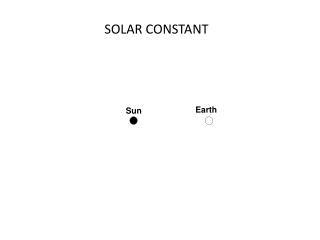DownloadDownload PresentationSOLAR CONSTANT

# SOLAR CONSTANT

Télécharger la présentation## SOLAR CONSTANT

- - - - - - - - - - - - - - - - - - - - - - - - - - - E N D - - - - - - - - - - - - - - - - - - - - - - - - - - -
##### Presentation Transcript

1. SOLAR CONSTANT

2. 1366 Wm-2

3. 1. 1366 W m-2 is radiated on a plane tangent to the Earth at all times – the “Solar Constant.” This amounts to only about one two-billionth of the power emitted from the Sun!

4. 2. This energy falls over a circular area of (π r2) square meters, where r is the radius of the earth, to give 1366πr2 total Watts of energy. 1. 1366 W m-2) is radiated on a plane tangent to the Earth at all times – the “Solar Constant.” This amounts to only about one two-billionth of the power emitted from the Sun! r

5. 2. This energy falls over a circular area of (π r2) square meters, where r is the radius of the earth, to give 1366πr2 total Watts of energy. 1. 1366 W m-2 is radiated on a plane tangent to the Earth at all times – the “Solar Constant.” This amounts to only about one two-billionth of the power emitted from the Sun! Note to artist: Make this the Earth, with land and ocean, etc. Ideally, it should tilt on its axis an the same angle as the Earths on spread 02.05A. Note to artist: Make this the Earth, with land and ocean, etc. Ideally, it should tilt on its axis an the same angle as the Earths on spread 02.05A. r r r r 3. But these 1366 πr2Watts fall over the entire surface area of Earth. The surface area of any sphere is 4πr2, so 1366πr2 W / 4πr2 square meters, or 342 W m-2.

6. CAN THE SUN CHANGE ITS ENERGY OUTPUT?

7. Staisticallysignificant oscillations at about 11 years